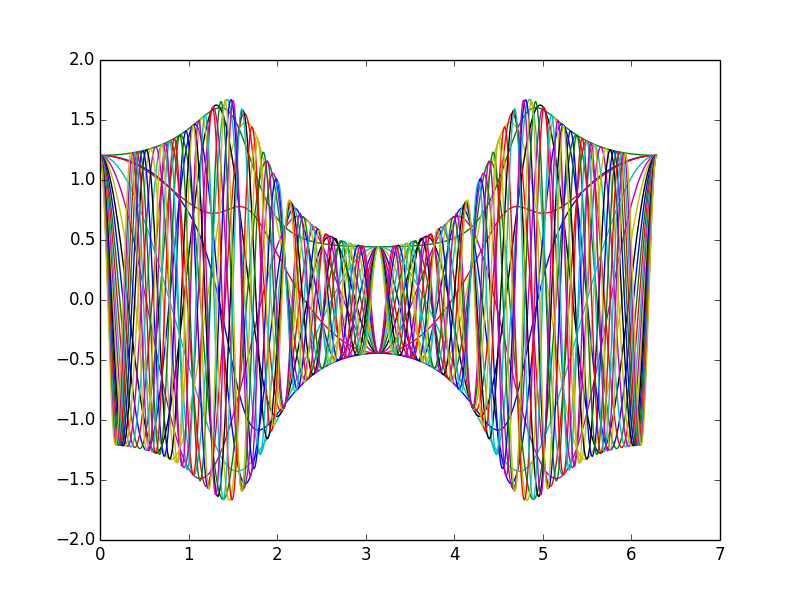# Fast plots - How do I do those?

I have no experience with Sage plots so I think I make something wrong. With Maple

plot([seq(Re(exp(I*t*(1-n))*(1+(exp(exp(I*t))-1)/(exp(2*exp(I*t))+1))),n=1..20)],t=0..2*Pi);


takes less than 1 second and it looks perfect.

With Sage (executed at SMC)

f = lambda x,n: (exp(i*x*(1-n))*(1+(exp(exp(i*x))-1)/(exp(2*exp(i*x))+1))).real()
bat = plot(f(x,0), (0,2*pi), color=Color(0,0,0), ymin=-1.6, ymax=1.6)
for n in sxrange(1,20,1):
bat += plot(f(x,n), (0,2*pi), rgbcolor=(n/2,n/5,n/3), ymin=-1.6, ymax=1.6)
bat


takes longer than the lifespan of Methuselah. How can I speed things up?

edit retag close merge delete

Sort by » oldest newest most votedA possibility would be to use matplotlib+numpy. For example:

import numpy as np
import matplotlib.pyplot as plt

def get_f( n ):
i=np.complex(0,1)
def func(t):
return np.exp(i*t*(1-n))*(1+(np.exp(np.exp(i*t))-1)/(np.exp(2*np.exp(i*t))+1))
return func

def mat_plot():
t = np.arange(0.,2*np.pi,0.01)
for i in range(20):
func = get_f(i)
ft = func(t)
plt.plot(t,np.real(ft))
plt.show()
plt.clf()


Takes only 0.5s at SMC (and 234ms on my laptop). Sample output:more

1

Excellent, thanks! I call this plot Bernoulli's batman. If you want to know why you can go here: http://luschny.de/math/zeta/Bernoulli...

@Peter Luschny Nice! Are you planning on translating the source on the appendix to Sagemath?

Yes, I will add also a Sage version and add your graphic script, if you allow. The page is still under construction and more things might come.

As long as it preserves the same CC license I'm fine. I'll try to tweak the code a bit more.